# Polynomials

Polynomials are a particular class of expressions that are simple enough to have many properties that can be analyzed. In particular, the key concepts of calculus: limits, continuity, derivatives, and integrals are all relatively trivial for polynomial functions. However, polynomials are flexible enough that they can be used to approximate a wide variety of functions. Indeed, though we don't pursue this, we mention that Julia's ApproxFun package exploits this to great advantage.

Here we discuss some vocabulary and basic facts related to polynomials and show how the add-on SymPy package can be used to model polynomial expressions within SymPy.

For our purposes, a monomial is simply a non-negative integer power of $x$ (or some other indeterminate symbol) possibly multiplied by a scalar constant. For example, $5x^4$ is a monomial, as are constants, such as $-2=-2x^0$ and the symbol itself, as $x = x^1$. In general, one may consider restrictions on where the constants can come from, and consider more than one symbol, but we won't pursue this here, restricting ourselves to the case of a single variable and real coefficients.

A polynomial is a sum of monomials. After combining terms with same powers, a non-zero polynomial may be written uniquely as:

$a_n x^n + a_{n-1}x^{n-1} + \cdots a_1 x + a_0, \quad a_n \neq 0$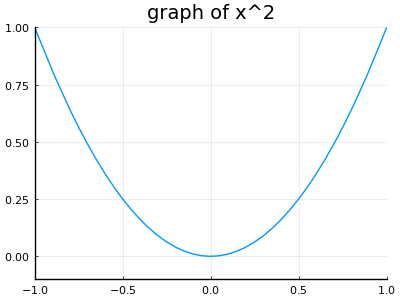Polynomials of varying even degrees over [-1,1].

The numbers $a_0, a_1, \dots, a_n$ are the coefficients of the polynomial. With the convention that $x=x^1$ and $1 = x^0$, the monomials above have their power match their coefficient's index, e.g., $a_ix^i$. Outside of the coefficient $a_n$, the other coefficients may be negative, positive, or $0$. Except for the zero polynomial, the largest power $n$ is called the degree. The degree of the zero polynomial is typically not defined or defined to be $-1$, so as to make certain statements easier to express. The term $a_n$ is called the leading coefficient. When the leading coefficient is $1$, the polynomial is called a monic polynomial. The monomial $a_n x^n$ is the leading term.

For example, the polynomial $-16x^2 - 32x + 100$ has degree $2$, leading coefficient $-16$ and leading term $-16x^2$. It is not monic, as the leading coefficient is not 1.

Lower degree polynomials have special names: a degree $0$ polynomial ($a_0$) is a non-zero constant, a degree 1 polynomial ($a_0+a_1x$) is called linear, a degree $2$ polynomial is quadratic, and a degree $3$ polynomial is called cubic.

## Linear polynomials

A special place is reserved for polynomials with degree 1. These are linear, as their graphs are straight lines. The general form,

$a_1 x + a_0, \quad a_1 \neq 0,$

is often written as $mx + b$, which is the slope-intercept form. The slope of a line determines how steeply it rises. The value of $m$ can be found from two points through the well-known formula:

$m = \frac{y_1 - y_0}{x_1 - x_0} = \frac{\text{rise}}{\text{run}}$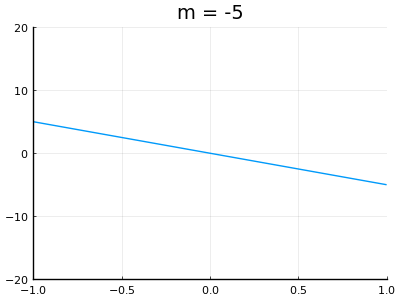Graphs of y = mx for different values of m

The intercept, $b$, comes from the fact that when $x=0$ the expression is $b$. That is the graph of the function $f(x) = mx + b$ will have $(0,b)$ as a point on it.

More generally, we have the point-slope form of a line, written as a polynomial through

$y_0 + m \cdot (x - x_0).$

The slope is $m$ and the point $(x_0, y_0)$. Again, the line graphing this as a function of $x$ would have the point $(x_0,y_0)$ on it and have slope $m$. This form is more useful in calculus, as the information we have convenient is more likely to be related to a specific value of $x$, not the special value $x=0$.

Thinking in terms of transformations, this looks like the function $f(x) = x$ (whose graph is a line with slope 1) stretched in the $y$ direction by a factor of $m$ then shifted right by $x_0$ units, and then shifted up by $y_0$ units. When $m>1$, this means the line grows faster. When $m< 0$, the line $f(x)=x$ is flipped through the $x$-axis so would head downwards, not upwards like $f(x) = x$.

## Symbolic math in Julia

The indeterminate value x (or some other symbol) in a polynomial, is like a variable in a function and unlike a variable in Julia. Variables in Julia are identifiers, just a means to look up a specific, already determined, value. Rather, the symbol x is not yet determined, it is essentially a place holder for a future value. Although we have seen that Julia makes it very easy to work with mathematical functions, it is not the case that base Julia makes working with expressions of algebraic symbols easy. This makes sense, Julia is primarily designed for technical computing, where numeric approaches rule the day. However, symbolic math can be used from within Julia with an add-on package.

Symbolic math programs include well-known ones like the commercial programs Mathematica and Maple. Mathematica powers the popular WolframAlpha website, which turns "natural" language into the specifics of a programming language. The open-source Sage project is an alternative to these two commercial giants. It includes a wide-range of open-source math projects available within its umbrella framework. (Julia can even be run from within the free service cloud.sagemath.com.) A more focused project for symbolic math, is the SymPy Python library. SymPy is also used within Sage. However, SymPy provides a self-contained library that can be used standalone within a Python session. That is great for Julia users, as the PyCall package glues Julia to Python in a seamless manner. This allows the Julia package SymPy to provide functionality from SymPy within Julia.

To use SymPy, we create symbolic objects to be our indeterminate symbols. The symbols function does this and is used like:

using CalculusWithJulia   # loads the SymPy package
using Plots
a,b,c = symbols("a,b,c")
x = symbols("x", real=true)

\begin{equation*}x\end{equation*}

The first use, shows that multiple symbols can be defined at once. The second shows the extra keyword argument real=true, which instructs SymPy to assume the x is real, as otherwise it assumes it is possibly complex. There are many other assumptions that can be made.

The macro @vars is like the second usage, only it does not need assignment, as the variable are created behind the scenes. This may be the easiest way to create symbolic values:

@vars h t

(h, t)


The SymPy package does two basic things:

• It imports some of the functionality provided by SymPy, including the ability to create symbolic variables.

• It overloads many Julia functions to work seamlessly with symbolic expressions. This makes working with polynomials quite natural.

To illustrate, using the just defined x, here is how we can create the polynomial $-16x^2 + 100$:

p = -16x^2 + 100

\begin{equation*}100 - 16 x^{2}\end{equation*}

That is, the expression is created just as you would create it within a function body. But here the result is still a symbolic object. We have assigned this expression to a variable p, and have not defined it as a function p(x). Mentally keeping the distinction between expressions and functions is very important.

The typeof function shows that p is of a symbolic type (Sym):

typeof(p)

Sym


We can mix and match symbolic objects. This command creates an arbitrary quadratic polynomial:

quad = a*x^2 + b*x + c

\begin{equation*}a x^{2} + b x + c\end{equation*}

Again, this is entered in a manner nearly identical to how we see such expressions typeset ($ax^2 + bx+c$), though we must remember to explicitly place the multiplication operator, as the symbols are not numeric literals.

We can apply many of Julia's mathematical functions and the result will still be symbolic:

sin(a*(x - b*pi) + c)

\begin{equation*}\sin{\left(a \left(- \pi b + x\right) + c \right)}\end{equation*}

Another example, might be the following combination:

quad + quad^2 - quad^3

\begin{equation*}a x^{2} + b x + c - \left(a x^{2} + b x + c\right)^{3} + \left(a x^{2} + b x + c\right)^{2}\end{equation*}

## Substitution: subs, replace

Algebraically working with symbolic expressions is straightforward. A different symbolic task is substitution. For example, replacing each instance of x in a polynomial, with, say, (x-1)^2. Substitution requires three things to be specified: an expression to work on, a variable to substitute, and a value to substitute in.

SymPy provides its subs function for this. This function is available in Julia, but it is easier to use notation reminiscent of function evaluation.

To illustrate, to do the task above for the polynomial $-16x^2 + 100$ we could have:

p = -16x^2 + 100
p(x => (x-1)^2)

\begin{equation*}100 - 16 \left(x - 1\right)^{4}\end{equation*}

This "call" notation takes pairs (designated by a=>b) where the left-hand side is the variable to substitute for, and the right-hand side the new value. The value to substitute can depend on the variable, as illustrated; be a different variable; or be a numeric value, such as $2$:

y = p(x=>2)

\begin{equation*}36\end{equation*}

The result will always be of a symbolic type, even if the answer is just a number:

typeof(y)

Sym


If there is just one free variable in an expression, the pair notation can be dropped:

p(4) # substitutes x=>4

\begin{equation*}-156\end{equation*}
##### Example

Suppose we have the polynomial $p = ax^2 + bx +c$. What would it look like if we shifted right by $E$ units and up by $F$ units?

@vars a b c E F
p = a*x^2 + b*x + c
p(x => x-E) + F

\begin{equation*}F + a \left(- E + x\right)^{2} + b \left(- E + x\right) + c\end{equation*}

And expanded this becomes:

expand(p(x => x-E) + F)

\begin{equation*}E^{2} a - 2 E a x - E b + F + a x^{2} + b x + c\end{equation*}

### Conversion of symbolic numbers to Julia numbers

In the above, we substituted 2 in for x to get y:

p = -16x^2 + 100
y = p(2)

\begin{equation*}36\end{equation*}

The value, $36$ is still symbolic, but clearly an integer. If we are just looking at the output, we can easily translate from the symbolic value to an integer, as they print similarly. However the conversion to an integer, or another type of number, does not happen automatically. If a number is needed to pass along to another Julia function, it may need to be converted. In general, conversions between different types are handled through various methods of convert. However, with SymPy, the N function will attempt to do the conversion for you:

N(y)

36


Conversion by N also works for other types of data, such as Rational and Float64. For getting more digits of accuracy, a precision can be passed to N. The following command will take the symbolic value for $\pi$, PI, and produce about 60 digits worth as a BigFloat value:

N(PI, 60)

3.141592653589793238462643383279502884197169399375105820974939


Conversion will fail if the value to be converted contains free symbols, as would be expected.

## Graphical properties of polynomials

Consider the graph of the polynomial x^5 - x + 1:

plot(x^5 - x + 1, -3/2, 3/2)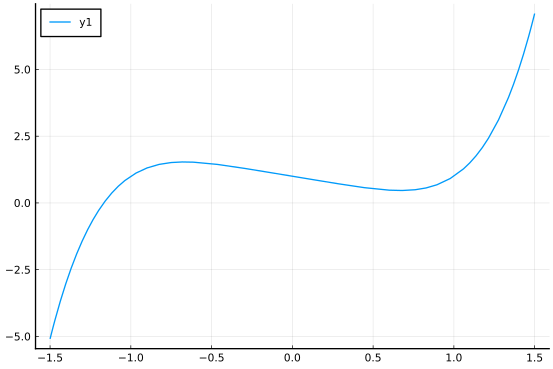(Plotting symbolic expressions is similar to plotting a function, in that the expression is passed in as the first argument. The expression must have only one free variable, as above, or an error will occur.)

This graph illustrates the key features of polynomial graphs:

• there may be values for x where the graph crosses the $x$ axis (real roots of the polynomial);

• there may be peaks and valleys (local maxima and local minima)

• except for constant polynomials, the ultimate behaviour for large values of $\lvert x\rvert$ is either both sides of the graph going to positive infinity, or negative infinity, or as in this graph one to the positive infinity and one to negative infinity. In particular, there is no horizontal asymptote.

To investigate this last point, let's consider the case of the monomial $x^n$. When $n$ is even, the following animation shows that larger values of $n$ have greater growth once outside of $[-1,1]$: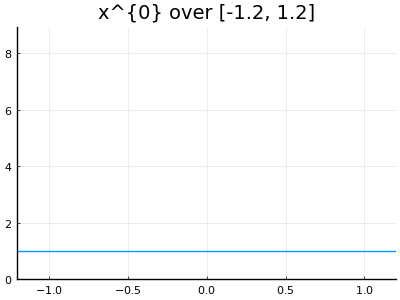Demonstration that $x^{10}$ grows faster than $x^8$, ... and $x^2$ grows faster than $x^0$ (which is constant).

Of course, this is expected, as, for example, $2^2 < 2^4 < 2^6 < \cdots$. The general shape of these terms is similar - $U$ shaped, and larger powers dominate the smaller powers as $\lvert x\rvert$ gets big.

For odd powers of $n$, the graph of the monomial $x^n$ is no longer $U$ shaped, but rather constantly increasing. This graph of $x^5$ is typical:

plot(x^5, -2, 2)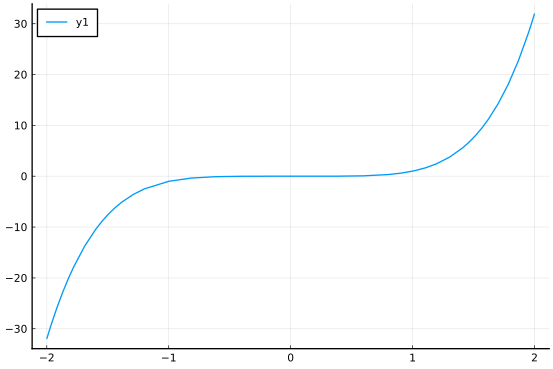Again, for larger powers the shape is similar, but the growth is faster.

### Leading term dominates

To see the roots and/or the peaks and valleys of a polynomial requires a judicious choice of viewing window, as ultimately the leading term will dominate the graph. The following animation of the graph of $(x-5)(x-3)(x-2)(x-1)$ illustrates. Subsequent images show a widening of the plot window until the graph appears U-shaped.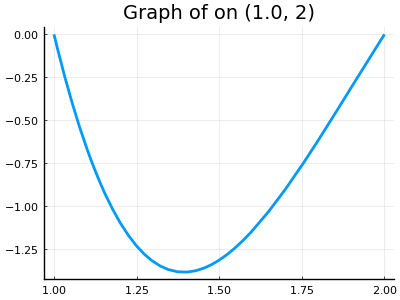The previous graph is highlighted in red. Ultimately the leading term ($x^4$ here) dominates the graph.

The leading term in the animation is $x^4$, so the graphic is U-shaped, were it an odd power, then the left and right sides would each head off to different signs of infinity.

To illustrate analytically why the leading term dominates, consider the polynomial $2x^5 - x + 1$ and then factor out the largest power, $x^5$, leaving a product:

$x^5 \cdot (2 - \frac{1}{x^4} + \frac{1}{x^5}).$

For large $\lvert x\rvert$, the last two terms in the product on the right get close to $0$, so this expression is basically just $2x^5$ - the leading term.

##### Example

Suppose $p = a_n x^n + \cdots + a_1 x + a_0$ with $a_n > 0$. Then by the above, eventually for large $x > 0$ we have $p > 0$, as that is the behaviour of $a_n x^n$. Were $a_n < 0$, then eventually for large $x>0$, $p < 0$.

Now consider the related polynomial, $q$, where we multiply $p$ by $x^n$ and substitute in $1/x$ for $x$. This is the "reversed" polynomial, as we see in this illustration for $n=2$:

p = a*x^2 + b*x + c
n = 2    # the degree of p
q = expand(x^n * p(x => 1/x))

\begin{equation*}a + b x + c x^{2}\end{equation*}

In particular, from the reversal, the behavior of $q$ for large $x$ depends on the sign of $x_0$. As well, due to the $1/x$, the behaviour of $q$ for large $x>0$ is the same as the behaviour of $p$ for small positive $x$. In particular if $a_n > 0$ but $a_0 < 0$, then p is eventually positive and q is eventually negative.

That is, if $p$ has $a_n > 0$ but $a_0 < 0$ then the graph of $p$ must cross the $x$ axis.

This observation is the start of Descartes' rule of signs, which counts the change of signs of the coefficients in p to say something about how many possible crossings there are of the $x$ axis by the graph of the polynomial $p$.

## Factoring polynomials

Among others, there are two common ways of representing a non-zero polynomial: either in an

• expanded form, as in $a_n x^n + a_{n-1}x^{n-1} + \cdots a_1 x + a_0, a_n \neq 0$; or

• in factored form, as in $a\cdot(x-r_1)\cdot(x-r_2)\cdots(x-r_n), a \neq 0$.

The latter writes $p$ as a product of linear factors, though this is only possible in general if we consider complex roots. With real roots only, then the factors are either linear or quadratic, as will be discussed later.

There are values to each representation. One value of the expanded form is that doing arithmetic with polynomials is much easier in expanded form. For example, adding polynomials just requires matching up the monomials of similar powers. As for the factored format, the one value is that it is easy to read off roots of the polynomial (values of $x$ where $p$ is $0$), as a product is $0$ only if a term is $0$, so any zero must be a zero of a factor. However, factored form has other advantages. For example, the polynomial $(x-1)^{1000}$ can be compactly represented using the factored form, but would require 1001 coefficients to store in expanded form. (As well, due to floating point differences, the two would evaluate quite differently as one would require over a 1000 operations to compute, the other just two.)

Translating from factored form to expanded form can be done by carefully following the distributive law of multiplication. For example, with some care it can be shown that:

$(x-1) \cdot (x-2) \cdot (x-3) = x^3 - 6x^2 +11x - 6.$

The SymPy function expand will perform these algebraic manipulations without fuss:

expand((x-1)*(x-2)*(x-3))

\begin{equation*}x^{3} - 6 x^{2} + 11 x - 6\end{equation*}

Factoring a polynomial is several weeks worth of lessons, as there is no one-size-fits-all algorithm to follow. There are some tricks that are taught: for example factoring differences of perfect squares, completing the square, the rational root theorem, $\dots$. But in general the solution is not automated. The SymPy function factor will find all rational factors (terms like $(qx-p)$), but will leave terms that do not have rational factors alone. For example:

factor(x^3 - 6x^2 + 11x -6)

\begin{equation*}\left(x - 3\right) \left(x - 2\right) \left(x - 1\right)\end{equation*}

Or

factor(x^5 - 5x^4 + 8x^3 - 8x^2 + 7x - 3)

\begin{equation*}\left(x - 3\right) \left(x - 1\right)^{2} \left(x^{2} + 1\right)\end{equation*}

But will not factor things that are not hard to see:

x^2 - 2

\begin{equation*}x^{2} - 2\end{equation*}

The factoring $(x-\sqrt{2})\cdot(x + \sqrt{2})$ is not found, as $\sqrt{2}$ is not rational.

(For those, it may be possible to solve to get the roots, which can then be used to produce the factored form.)

### Polynomial functions and polynomials.

Our definition of a polynomial is in terms of algebraic expressions which are easily represented by SymPy objects, but not objects from base Julia. (Though there are the Polynomials package and the AbstractAlbegra package for representing polynomials.)

However, polynomial functions are easily represented by Julia, for example,

f(x) = -16x^2 + 100

f (generic function with 1 method)


The distinction is subtle, the expression is turned into a function just by adding the f(x) = preface. But to Julia there is a big distinction. The function form never does any computation until after a value of $x$ is passed to it. Whereas symbolic expressions can be manipulated quite freely before any numeric values are specified.

It is easy to create a symbolic expression from a function - just evaluate the function on a symbolic value:

f(x)

\begin{equation*}100 - 16 x^{2}\end{equation*}

This is easy - but can also be confusing. The function object is f, the expression is f(x) - the function evaluated on a symbolic object. SymPy provides an interface for a few commonly used functions so that either will work. One such is plot, either plot(f, a, b) or plot(f(x),a, b) will produce the same plot with Plots.

Symbolic polynomial expressions can be evaluated using the same syntax as a function call:

p = -16*x^2 + 100
p(2)

\begin{equation*}36\end{equation*}

If desired, such expressions can be converted into a function using Julias convert function.

## Questions

###### Question

Let $p$ be the polynomial $3x^2 - 2x + 5$.

What is the degree of $p$?

What is the leading coefficient of $p$?

The graph of $p$ would have what $y$-intercept?

Is $p$ a monic polynomial?

Is $p$ a quadratic polynomial?

The graph of $p$ would be $U$-shaped?

What is the leading term of $p$?

###### Question

Let $p = x^3 - 2x^2 +3x - 4$.

What is $a_2$, using the standard numbering of coefficient?

What is $a_n$?

What is $a_0$?

###### Question

The linear polynomial $p = 2x + 3$ is written in which form:

###### Question

The polynomial p is defined in Julia as follows:

\begin{equation*}64 - 16 x^{2}\end{equation*}

What command will return the value of the polynomial when $x=2$?

###### Question

In the large, the graph of $p=x^{101} - x + 1$ will

###### Question

In the large, the graph of $p=x^{102} - x^{101} + x + 1$ will

###### Question

In the large, the graph of $p=-x^{10} + x^9 + x^8 + x^7 + x^6$ will

###### Question

Use SymPy to factor the polynomial $x^{11} - x$. How many factors are found?

###### Question

Use SymPy to factor the polynomial $x^{12} - 1$. How many factors are found?

###### Question

What is the monic polynomial with roots $x=-1$, $x=0$, and $x=2$?

###### Question

Use expand to expand the expression ((x-h)^3 - x^3) / h where x and h are symbolic constants. What is the value: